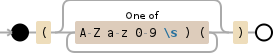# How to find all possible regex matches in python?

I am trying to find all possible word/tag pairs or other nested combinations with python and its regular expressions.

``````sent = '(NP (NNP Hoi) (NN Hallo) (NN Hey) (NNP (NN Ciao) (NN Adios)))'

def checkBinary(sentence):
n = re.findall("\([A-Za-z-0-9\s\)\(]*\)", sentence)
print(n)

checkBinary(sent)

Output:
['(NP (NNP Hoi) (NN Hallo) (NN Hey) (NNP (NN Ciao) (NN Adios)))']
``````

looking for:

``````['(NP (NNP Hoi) (NN Hallo) (NN Hey) (NNP (NN Ciao) (NN Adios)))',
'(NNP Hoi)',
'(NN Hallo)',
'(NN Hey)',
'(NN Ciao)',
``````

I think the regex formula could find the nested parenthesis word/tag pairs aswell but it doesn't return them. How should I do this?

• It's "Binary"... – ThiefMaster May 14 '14 at 21:14

it's actually not possible to do this by using regular expressions, because regular expressions express a language defined by a regular grammar that can be solved by a non finite deterministic automaton, where matching is represented by states ; then to match nested parenthesis, you'd need to be able to match an infinite number of parenthesis and then have an automaton with an infinite number of states.

To be able to cope with that, we use what's called a push-down automaton, that is used to define the context free grammar.So if your regex does not match nested parenthesis, it's because it's expressing the following automaton and does not match anything on your input:Play with it

As a reference, please have a look at MIT's courses on the topic:

So one of the ways to parse your string efficiently, is to build a grammar for nested parenthesis (`pip install pyparsing` first):

``````>>> import pyparsing
>>> strings = pyparsing.Word(pyparsing.alphanums)
>>> parens  = pyparsing.nestedExpr( '(', ')', content=strings)
>>> parens.parseString('(NP (NNP Hoi) (NN Hallo) (NN Hey) (NNP (NN Ciao) (NN Adios)))').asList()
[['NP', ['NNP', 'Hoi'], ['NN', 'Hallo'], ['NN', 'Hey'], ['NNP', ['NN', 'Ciao'], ['NN', 'Adios']]]]
``````

N.B.: there exists a few regular expressions engines that do implement nested parenthesis matching using the push down. The default python `re` engine is not one of them, but an alternative engine exists, called `regex` (`pip install regex`) that can do recursive matching (which makes the re engine context free), cf this code snippet:

``````>>> import regex
>>> res = regex.search(r'(?<rec>\((?:[^()]++|(?&rec))*\))', '(NP (NNP Hoi) (NN Hallo) (NN Hey) (NNP (NN Ciao) (NN Adios)))')
>>> res.captures('rec')
['(NNP Hoi)', '(NN Hallo)', '(NN Hey)', '(NN Ciao)', '(NN Adios)', '(NNP (NN Ciao) (NN Adios))', '(NP (NNP Hoi) (NN Hallo) (NN Hey) (NNP (NN Ciao) (NN Adios)))']
``````
• CS at it's essence. +1 – PepperoniPizza May 14 '14 at 12:34
• Oh modern regex could match this kind of data. Read about recursive patterns and/or balancing groups. Reference – HamZa May 14 '14 at 13:08
• indeed, and I even suggest a recursive pattern solution at the end. Though, per definition, those are not regular expressions anymore. – zmo May 14 '14 at 13:09
• @FarhadAliNoo Per definition of regular expressions in formal theory, a regular expression is implementing a regular grammar. The engines implementing non-regular expressions should take a different name, like nregex (for non-regular expressions) or cfex (context-free expressions)… Even though those abilities have been implemented in regex engines, calling them regex is like calling a plane "a car that can fly". So maybe someone should make an article on HN to complain about that :-) – zmo May 14 '14 at 14:27
• let's blame the Perl people! :-D – zmo May 14 '14 at 14:36

Regular expressions used in modern languages DO NOT represent regular languages. zmo is right in saying that regular languages in Language Theroy are represented by finite state automata but the regular expressions that use any sort of backtracking like those with capturing groups, lookarounds and etc that are used in modern languages CANNOT be represented by FSAs known in Language Theory. How can you represent a pattern like (\w+)\1 with a DFA or even and NFA?

The regular expression you are looking for can be something like this(only matches to two levels):

``````(?=(\((?:[^\)\(]*\([^\)]*\)|[^\)\(])*?\)))
``````

I tested this on http://regexhero.net/tester/

The matches are in the captured groups:

1: (NP (NNP Hoi) (NN Hallo) (NN Hey) (NNP (NN Ciao) (NN Adios))

1: (NNP Hoi)

1: (NN Hallo)

1: (NN Hey)

1: (NNP (NN Ciao) (NN Adios))

1: (NN Ciao)

• I believe @zmo talked about modern regular expression in the ending `NB` part (and gave an example using recursion). Also be careful, your expression doesn't go deeper than two level of nesting: your first match is missing the closing parenthesis. – Robin May 14 '14 at 13:37